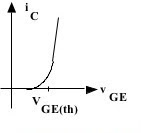## Page

### Characteristics of IGBT

The circuit of figure circuit diagram shows the various parameters pertaining in the IGBT characteristics.

Static I-V or output characteristics of an IGBT (n channel type) show the plot of conduct collector current Iversus collector-emitter voltage VCE for various value of gate emitter voltages VGE1, VGE2 etc. These characteristics are shown in figure static I-V characteristics.In the forward directions, the shape of the output characteristics is similar to that of BJT. But here the controlling parameter is gate emitter voltage VGE because IGBT is a voltage-controlled device is when the device is of junction Jto block the forward voltage and in case reverse voltage appears across collector and emitter junction J1 blocks it. In static I-V characteristics, VRM is the maximum reverse breakdown voltage.

The transfer characteristics of an IGBT is a plot of collector current IC vs gate emitter voltage VGE as shown in figure transfer characteristics. This characteristic is identical to that of power MOSFET when VGE is less than the threshold voltage VGET, IGBT is in the offset.

## Output Characteristics :

• These connection are made as shown in the circuit diagram.
• Now increase the VCE voltage to the maximum position.
• When slowly increase the voltage across the VGE at certain voltage IGBT is turned on stop varying the voltage at that point.
• Then reduce the voltage  VCE to the minimum position.
• Now vary VCE voltage slowly, correspondingly note down the VCE and IC readings and plot the graph.

### Transfer characteristic :

• These connections are made as shown in the circuit diagram.
• In these characteristics when increase the voltage  VCE voltage to the maximum position.
• When slowly increase increase the voltage across VGE and note down the voltage VGE and current IC value and plot graph.
The circuit of figure circuit diagram shows the various parameters pertaining in the IGBT characteristics.

Static I-V or output characteristics of an IGBT (n channel type) show the plot of conduct collector current Iversus collector-emitter voltage VCE for various value of gate emitter voltages VGE1, VGE2 etc. These characteristics are shown in figure static I-V characteristics.In the forward directions, the shape of the output characteristics is similar to that of BJT. But here the controlling parameter is gate emitter voltage VGE because IGBT is a voltage-controlled device is when the device is of junction Jto block the forward voltage and in case reverse voltage appears across collector and emitter junction J1 blocks it. In static I-V characteristics, VRM is the maximum reverse breakdown voltage.

The transfer characteristics of an IGBT is a plot of collector current IC vs gate emitter voltage VGE as shown in figure transfer characteristics. This characteristic is identical to that of power MOSFET when VGE is less than the threshold voltage VGET, IGBT is in the offset.

## Output Characteristics :

• These connection are made as shown in the circuit diagram.
• Now increase the VCE voltage to the maximum position.
• When slowly increase the voltage across the VGE at certain voltage IGBT is turned on stop varying the voltage at that point.
• Then reduce the voltage  VCE to the minimum position.
• Now vary VCE voltage slowly, correspondingly note down the VCE and IC readings and plot the graph.

### Transfer characteristic :

• These connections are made as shown in the circuit diagram.
• In these characteristics when increase the voltage  VCE voltage to the maximum position.
• When slowly increase increase the voltage across VGE and note down the voltage VGE and current IC value and plot graph.